﻿ 基于LSTM模型模拟安康水库洪水过程

# 基于LSTM模型模拟安康水库洪水过程Simulation of Ankang Reservoir Inflow Based on LSTM Model

Abstract: The underlying surface conditions have changed greatly in the Ankang river basin due to human activities, and the error of the flood forecasting system is relatively large. Considering that hydrological data is a complex time series, it is difficult for ordinary hydrological models to capture its changing laws. LSTM (Long-short term memory), as a learning network with memory ability, can learn the complex and chan-geable time of hydrological data well by continuously inputting new data and learning the main features and changes of time series sequence. The LSTM network model is used to simulate the flood process of Ankang reservoir and compare with the Xinanjiang model. The adaptability of LSTM model in reservoir flood prediction is also discussed.

1. 概述

1.1. 研究背景

1.2. 研究对象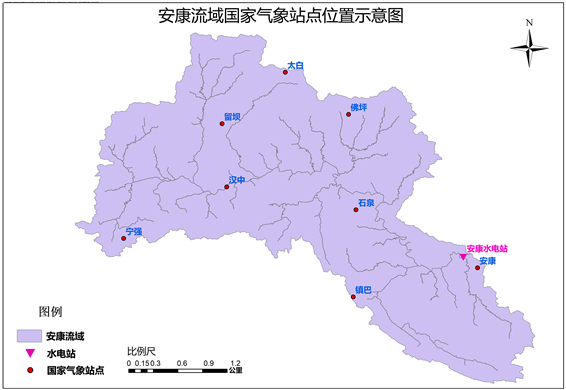Figure 1. Location map of the meteorological and hydrological stations in the Ankang River basin

2. 模型介绍

2.1. LSTM模型

Hochreiter和Schmidhuber引入长短期记忆(LSTM)神经网络，是一种特殊的循环神经网络(Recurrent Neural Network, RNN) ，其结构如图2所示，许多人进行了改进和普及，解决了RNN的长期记忆能力上的缺陷。LSTM神经网络通过在神经网络的隐藏层(Hidden layer)中引入存储单元(Memory cell)，即输入门(Input gate)、忘记门(Forget gate)、内部回馈连结(Self-recurrent connection)、和输出门(Output gate)来选择记忆当前信息或遗忘过去记忆信息，以增强神经网络的长期记忆能力 。

${h}_{t}=\delta \left(U{x}_{t}+W{h}_{t-1}+b\right)$ (1)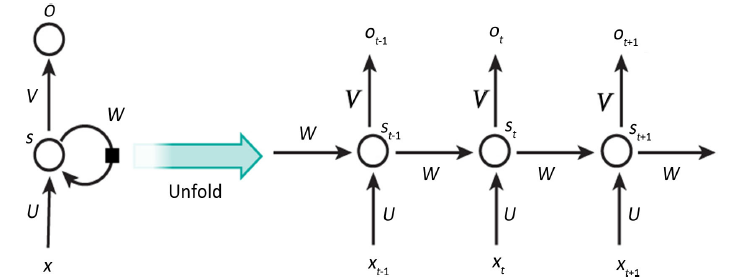Figure 2. Recurrent neural network structure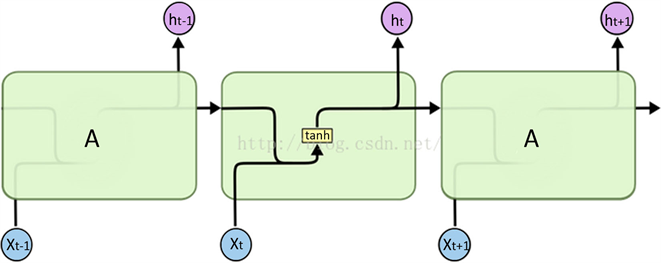Figure 3. Complete RNN unit diagram

LSTM神经网络是一种在RNN (如图3)基础上改进的递归神经网络，用来解决RNN使用过程中所产生的梯度消失、梯度爆炸以及缺乏长期记忆能力等问题。Bengio等  已经表明，传统的RNN几乎不能记住长度超过10的序列，对于日流量模拟，这就意味着我们只能使用最近10天的气象数据作为输入来预测第二天的流量。考虑到地下水，积雪甚至冰川储存等集水区的记忆，这段时间太短，降水和排放之间的滞后时间达到几年。为解决RNN在计算过程中出现梯度消失的问题，LSTM神经网络在原有的RNN神经网络结构的基础上，将隐含层的RNN计算单元换成结构更加复杂的LSTM计算单元，更新了计算网络的节点。LSTM神经网络通过设置多个不同门的开关实现时间轴上的记忆和遗忘功能，解决梯度消失问题，在每个LSTM神经网络计算单元设置3个门控制器，分别为输入门、输出门和遗忘门，形成一个新的计算单元。输入门控制特征值的信息输入，遗忘门控制计算单元状态信息的保留，输出门控制信息输出。

LSTM神经网络的构建思想是在RNN网络的基础上，将RNN中每个隐藏计算单元换成具有记忆功能的计算单元。LSTM层的计算可以表示如下(若干个计算单元组成一个LSTM层)：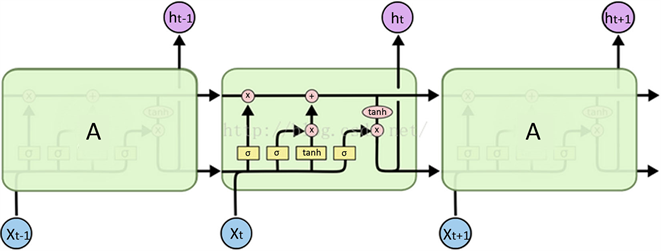Figure 4. Complete LSTM unit diagram

${g}^{\left(t\right)}=\varphi \left({W}_{gx}{x}^{\left(t\right)}+{W}_{ih}{h}^{\left(t-1\right)}+{b}_{g}\right)$ (2)

${i}^{\left(t\right)}=\sigma \left({W}_{ix}{x}^{\left(t\right)}+{W}_{ih}{h}^{\left(t-1\right)}+{b}_{i}\right)$ (3)

${f}^{\left(t\right)}=\sigma \left({W}_{fx}{x}^{\left(t\right)}+{W}_{fh}{h}^{\left(t-1\right)}+{b}_{f}\right)$ (4)

${o}^{\left(t\right)}=\sigma \left({W}_{ox}{x}^{\left(t\right)}+{W}_{oh}{h}^{\left(t-1\right)}+{b}_{o}\right)$ (5)

${h}^{\left(t\right)}={s}^{\left(t\right)}$ (6)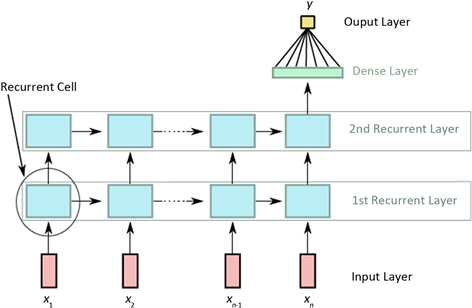Figure 5. LSTM calculation flow chart

2.2. 新安江模型

3. 实例计算与分析

3.1. 模型评价指标

$NS=1-\frac{{\sum }_{t=1}^{n}{\left({Q}_{ot}-{Q}_{pt}\right)}^{2}}{{\sum }_{t=1}^{n}{\left({Q}_{ot}-\stackrel{¯}{{Q}_{o}}\right)}^{2}}$ (7)

3.2. LSTM模拟计算结果

LSTM神经网络需要构建多层复杂网络结构，并且选取不同特征值来适配最优模拟目标结果，本文在尝试多种网络结构以及不同的特征值后，最终选取双层LSTM网络结构，选取时间、降雨、前一天入库流量作为特征值，模拟安康水库的入库洪水过程。

3.3. 新安江模拟计算结果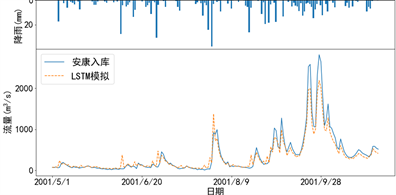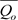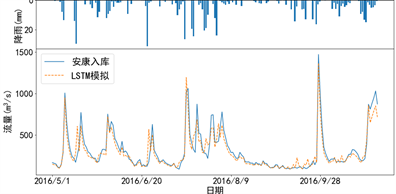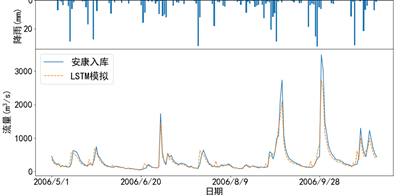Figure 6. Flood seasonal runoff hydrograph simulated by LSTM modelTable 1. Calibrated parameters of Xinanjiang model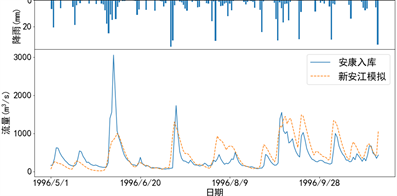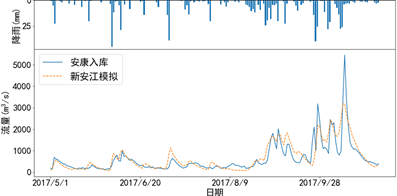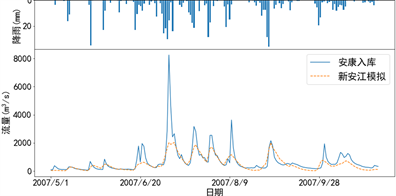Figure 7. Flood seasonal runoff hydrograph simulated by Xinanjiang model

3.4. 结果对比分析Table 2. Comparison of annual and flood seasonal Nash efficiency coefficients simulated by LSTM model and Xinanjiang model

4. 结论

1) LSTM模型对安康水库洪水过程模拟时，仅使用降雨与径流的历史过程，相对于新安江模型在一定程度上更简便，模拟效果也明显优于新安江模型。

2) 在日洪水过程模拟的基础上，进一步研究LSTM模型在时段洪水过程的模拟，以便将LSTM模型应用于安康水库实时洪水预报。

 武连洲, 白涛, 哈燕萍, 等. 水文序列变异对水库调度运行的影响研究[J]. 水资源与水工程学报, 2016, 27(4): 88-92. WU Lianzhou, BAI Tao, HA Yanping, et al. Effect of hydrological sequence variation on operation reservoir. Journal of Water Resources & Water Engineering, 2016, 27(4): 88-92. (in Chinese)

 杨光, 郭生练, 陈柯兵, 等. 基于决策因子选择的梯级水库多目标优化调度规则研究[J]. 水利学报, 2017, 48(8): 914-923. YANG Guang, GUO Shenglian, CHEN Kebing, et al. Multi-objective cascade reservoir optimal operation rules based on decision factor selection. Journal of Hydraulic Engineering, 2017, 48(8): 914-923. (in Chinese)

 冯钧, 潘飞. 一种LSTM-BP多模型组合水文预报方法[J]. 计算机与现代化, 2018(7): 82-85. FENG Jun, PAN Fei. A hydrologic forecast method based on LSTM-BP. Computer and Modernization, 2018(7): 82-85. (in Chinese)

 周研来, 郭生练, 张斐章, 等. 人工智能在水文预报中的应用研究[J]. 水资源研究, 2019(1): 1-12. ZHOU Yanlai, GUO Shenglian, CHANG Fi-John, et al. Hydrological forecasting using artificial intelligence techniques. Journal of Water Resources Research, 2019(1): 1-12. (in Chinese)

 陈卓, 孙龙祥. 基于深度学习LSTM网络的短期电力负荷预测方法[J]. 电子技术(上海), 2018, 47(1): 39-41. CHEN Zhuo, SUN Longxiang. Short-term electrical load forecasting based on deep learning LSTM networks. Electronics Design & Application, 2018, 47(1): 39-41. (in Chinese)

 BENGIO, Y., SIMARD, P. and FRASCONI, P. Learning long-term dependencies with gradient descent is difficult. IEEE transactions on neural networks, 1994, 5(2): 157-166.
https://doi.org/10.1109/72.279181

 HOCHREITER, S., SCHMIDHUBER, J. Long short-term memory. Neural Computation, 1997, 9(8): 1735-1780.
https://doi.org/10.1162/neco.1997.9.8.1735

 江衍铭, 张建全, 明焱. 集合神经网络的洪水预报[J]. 浙江大学学报(工学版), 2016, 50(8): 1471-1478. CHIANG Yenming, ZHANG Jianquan, MING Yan. Flood forecasting by ensemble neural networks. Journal of Zhejiang Uni-versity (Engineering Science), 2016, 50(8): 1471-1478. (in Chinese)

 钱承萍, 黄川友. 新安江三水源模型与水箱模型在清江流域上的应用与比较[J]. 西北水电, 2013(2): 4-7. QIAN Chengping, HUANG Chuanyou. Application and comparison of Xinanjiang model and Tank model in Qingjiang catch-ment Northwest Hydropower, 2013(2): 4-7. (in Chinese)

 赵人俊, 王佩兰. 新安江模型参数的分析[J]. 水文, 1988(6): 4-11. ZHAO Renjun, WANG Peilan. Analysis of Xinanjiang model parameters. Journal of China Hydrology, 1988(6): 4-11. (in Chi-nese)

 刘佩瑶, 郝振纯, 王国庆, 等. 新安江模型和改进BP 神经网络模型在闽江水文预报中的应用[J]. 水资源与水工程学报, 2017, 28(1): 40-44. LIU Peiyao, HAO Zhenchun, WANG Guoqing, et al. Application of Xinanjiang model and the improved BP neural network model in hydrological forecasting of the Min River. Journal of Water Resources and Water Engineering, 2017, 28(1): 40-44. (in Chinese)

Top